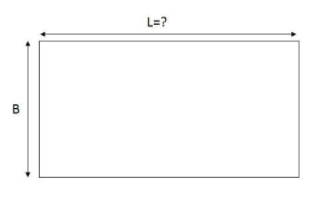QuestionAnswers

# The length of a rectangular hall is 4 meters less than 3 times the breadth of the hall. What is the length, if the breath is b metre?Hint: In this type of question first, we know about the perimeter of a triangle.
Perimeter of triangle =2(l+b)
Where l is the length of the rectangle
B is the breadth of the triangle
Here, the breadth is given and length is 3 × Breadth – 4
Now, we can proceed to the solution.

Complete step by step solution: First, we know about the breadth of a rectangular hall.As we know, the rectangle has 4 vertices and 4 edges and the internal angle is 90°.
In Euclidean plane geometry, a rectangle is a quadrilateral with four right angles.
The area of the rectangle is length × width and perimeter of the rectangle is 2 × (length + width)
It can also be defined as an equiangular quadrilateral, since equiangular means that all of its angles are equal.
According to the question,
Given that,
The breadth of the hall = b m
Now,
we find the length of the rectangular hall according to given condition, then,
length of the hall = 3 × (Breadth of hall) – 4
= 3 × b – 4
= 3b – 4
∴ length of hall = (3b – 4) meters.

Note: For solving this type of question, first, we know about the perimeter of triangle and area of a triangle that is The area of a rectangle is length × width and perimeter of a rectangle is 2 × (length + width)
It can also be defined as an equiangular quadrilateral, since equiangular means that all of its angles are equal.
View Notes
CBSE Class 8 Maths Chapter 4 - Practical Geometry FormulasGeometry Formulas For Class 8The Difference Between an Animal that is A Regulator and One that is A ConformerRelation Between the Length of a Given Wire and Tension for Constant Frequency Using SonometerWhere There is a Will There is a Way EssayWhat is the full form of phd?Measurement of Length DistanceThe Making of a ScientistChanging the Period of a PendulumTable of 4 - Multiplication Table of 4Important Questions for CBSE Class 8 Maths Chapter 4 - Practical GeometryImportant Questions for CBSE Class 8 Science Chapter 4 - Materials: Metals and Non-MetalsImportant Questions for CBSE Class 8 English Honeydew Chapter 4 - Bepin Choudhury’s Lapse of MemoryImportant Questions for CBSE Class 6 English A Pact with The Sun Chapter 8 - A Pact with the SunImportant Questions for CBSE Class 8 Social Science Our Past 3 Chapter 4 - Tribals, Dikus and the Vision of a Golden AgeCBSE Class 8 Science Reaching The Age of Adolescence WorksheetsImportant Questions for CBSE Class 8 English It So Happened Chapter 4 - The Treasure WithinImportant Questions for CBSE Class 8 Social Science Resources and Development Chapter 4 - AgricultureImportant Questions for CBSE Class 8 Social Science - Social and Political Life Chapter 4 - Understanding LawsImportant Questions for CBSE Class 6 English Honeysuckle Chapter 8 - A Game of ChanceCBSE Class 10 Hindi A Question Paper 2020Hindi A Class 10 CBSE Question Paper 2009Hindi A Class 10 CBSE Question Paper 2015Hindi A Class 10 CBSE Question Paper 2007Hindi A Class 10 CBSE Question Paper 2013Hindi A Class 10 CBSE Question Paper 2016Hindi A Class 10 CBSE Question Paper 2012Hindi A Class 10 CBSE Question Paper 2010Hindi A Class 10 CBSE Question Paper 2008Hindi A Class 10 CBSE Question Paper 2014NCERT Solutions for Class 8 Maths Chapter 4 Practical GeometryRD Sharma Class 8 Solutions Chapter 8 - Division of Algebraic Expressions (Ex 8.4) Exercise 8.4RD Sharma Class 8 Solutions Chapter 4 - Cube and Cube Roots (Ex 4.4) Exercise 4.4RS Aggarwal Solutions Class 8 Chapter-4 Cubes and Cube Roots (Ex 4D) Exercise 4.4NCERT Solutions for Class 8 Maths Chapter 4 Practical Geometry (EX 4.4) Exercise 4.4RD Sharma Class 8 Solutions Chapter 4 - Cube and Cube Roots (Ex 4.3) Exercise 4.3RD Sharma Class 7 Solutions Chapter 8 - Linear Equations in One Variable (Ex 8.4) Exercise 8.4RD Sharma Class 9 Solutions Chapter 8 - Lines and Angles (Ex 8.4) Exercise 8.4RS Aggarwal Solutions Class 8 Chapter-4 Cubes and Cube Roots (Ex 4C) Exercise 4.3RS Aggarwal Solutions Class 8 Chapter-4 Cubes and Cube Roots (Ex 4B) Exercise 4.2